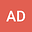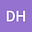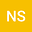Meir-Keeler type fixed point theorem and its application on integral equations
•••• +1
Anupam Das
Rajiv Gandhi University

Corresponding Author:math.anupam@gmail.com

Author ProfileNIPEN SAIKIA
Rajiv Gandhi University
Author ProfileNihar Kumar Mahato
PDPM Indian Institute of Information Technology Design and Manufacturing Jabalpur
Author Profile## Abstract

The aim of this article to propose some generalization of Meir-Keeler fixed point theorem with the help of an $\alpha$-admissible mapping. Further we prove the existence of solution of an infinite system of integral equations by using this generalized fixed point theorem involving measure of noncompactness in Banach space and illustrate the results with the help of an example. Finally, apply an iterative algorithm we find an approximate solution of an infinite system of integral equations.